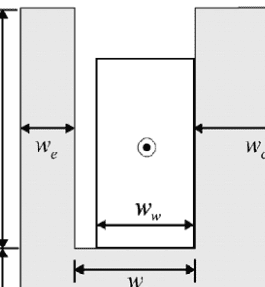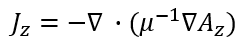# A question about current density in finite element analysis

• A
• JH_1870
In summary, the conversation is discussing the use of current density and Maxwell's equations when there are multiple current sources present. The individual is trying to determine which value to use and if the superposition principle needs to be applied. They are also considering the effects of ferromagnetic materials and how they may complicate the problem. The conversation suggests that this is a non-trivial problem and may require simplifying assumptions or may be very difficult to solve in the general case. Additional resources are recommended for further reading and understanding of the problem.

#### JH_1870

I know that if there is only one conductor providing the current density, then the current density can be used.But if you apply Maxwell's equation when there are multiple current sources, I don't know which value to use.

This is not an analysis using a tool, but a problem when I develop the code myself.

Should I calculate all the values for multiple independent sources and then add them up?Where J is the applied current density. Is it correct to use the applied current density when calculating the magnetic potential in the iron core?

And, when there are several applied current densities, is it necessary to apply the superposition principle to solve them?

This appears to be a very non-trivial problem that you are trying to solve. For problems with ferromagnetic materials, sometimes the geometry involved leads to simplifying assumptions, particularly in the case of transformers. You might find some good reading in this thread, along with some of the "links" that are referenced: https://www.physicsforums.com/threads/magnetic-flux-is-the-same-if-we-apply-the-biot-savart.927681/
For the general case of magnetic materials with currents in conductors, I think it may be a very difficult problem that you are trying to tackle.

Just an additional comment or two: This type of problem can not be solved as a perturbation with a small response and source generated from the ferromagnetic material. Instead, in many cases, the response of the ferromagnetic material may be many and many times larger than the original source from the free currents in the conductor. Perhaps others may also comment, but this is my take on this problem.

## 1. What is current density in finite element analysis?

Current density is a measure of the amount of electric current flowing through a unit area in a material. In the context of finite element analysis, it is used to determine the distribution of current within a conductive material.

## 2. How is current density calculated in finite element analysis?

Current density is calculated by dividing the total current passing through a given area by the cross-sectional area of the material. In finite element analysis, this is typically done using numerical methods to approximate the current distribution within the material.

## 3. Why is current density an important factor in finite element analysis?

Current density is important because it affects the behavior and performance of conductive materials. By accurately calculating and analyzing current density, we can better understand the flow of electricity within a material and predict potential issues or failures.

## 4. How does the mesh size affect current density in finite element analysis?

The mesh size, or the size of the individual elements in the finite element model, can have a significant impact on the accuracy of current density calculations. Smaller mesh sizes can provide more accurate results, but also require more computational resources and time.

## 5. Can current density be used to assess the quality of a conductive material?

Yes, current density can be used as a metric for the quality of a conductive material. A uniform and consistent current density distribution is indicative of a well-performing material, while irregularities or high concentrations of current can indicate potential weaknesses or defects.